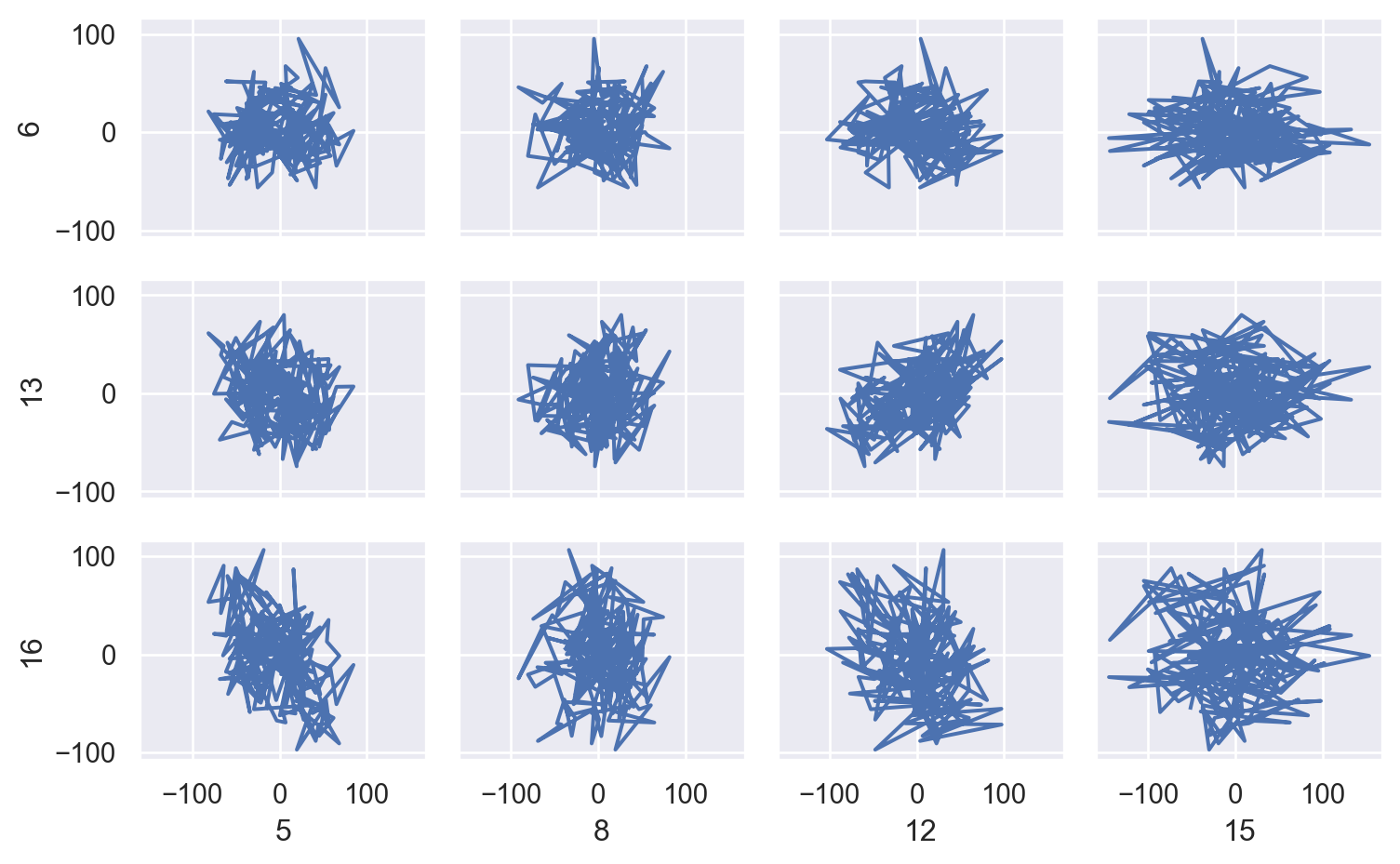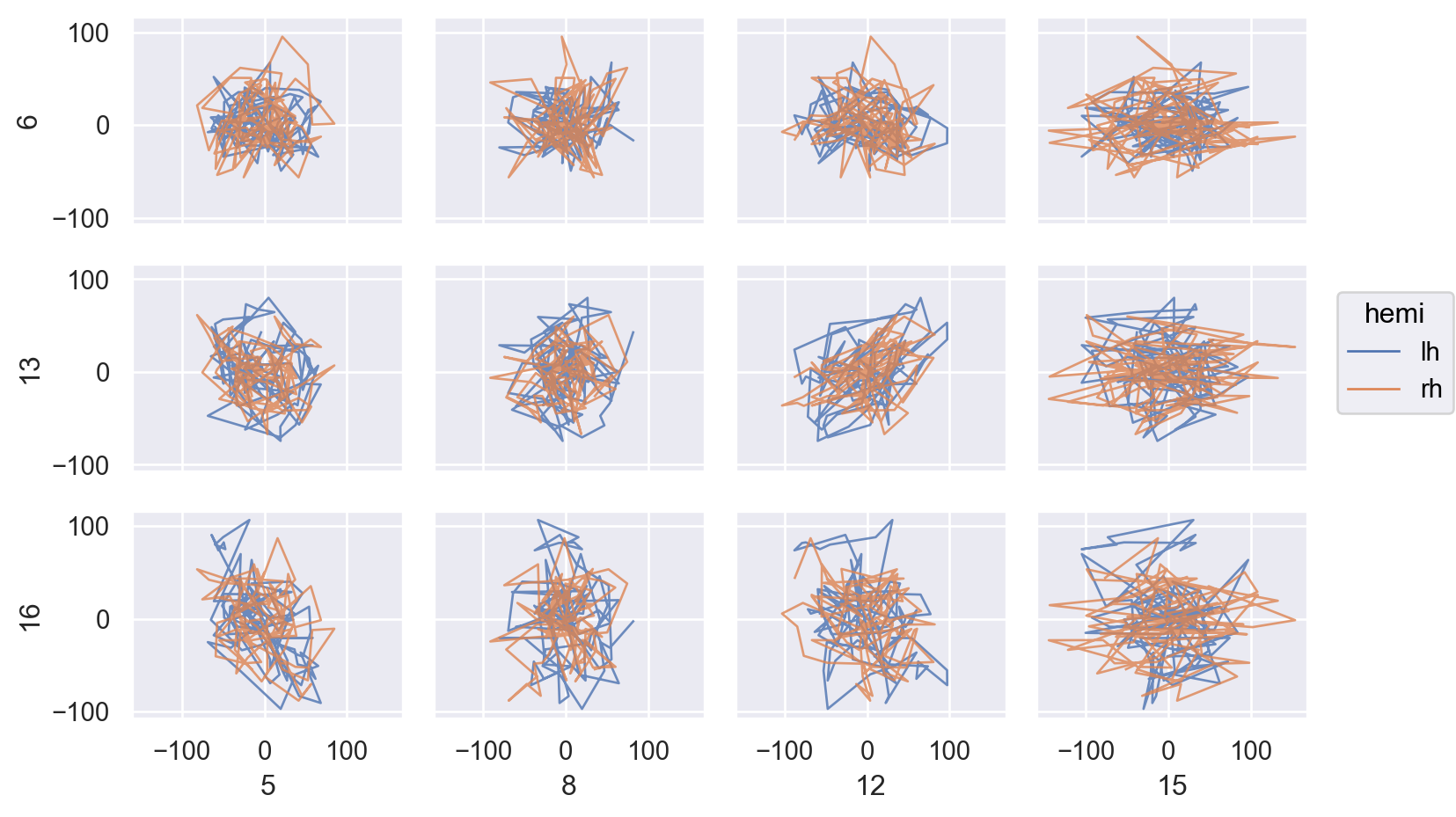# seaborn.objects.Paths#

class seaborn.objects.Paths(artist_kws=<factory>, color=<'C0'>, alpha=<1>, linewidth=<rc:lines.linewidth>, linestyle=<rc:lines.linestyle>)#

A faster but less-flexible mark for drawing many paths.

This mark defines the following properties:

`Path`

A mark connecting data points in the order they appear.

Examples

Unlike `Lines`, this mark does not sort observations before plotting, making it suitable for plotting trajectories through a variable space:

```p = (
so.Plot(networks)
.pair(
x=["5", "8", "12", "15"],
y=["6", "13", "16"],
)
.layout(size=(8, 5))
.share(x=True, y=True)
)The mark has the same set of properties as `Lines`:
```p.add(so.Paths(linewidth=1, alpha=.8), color="hemi")